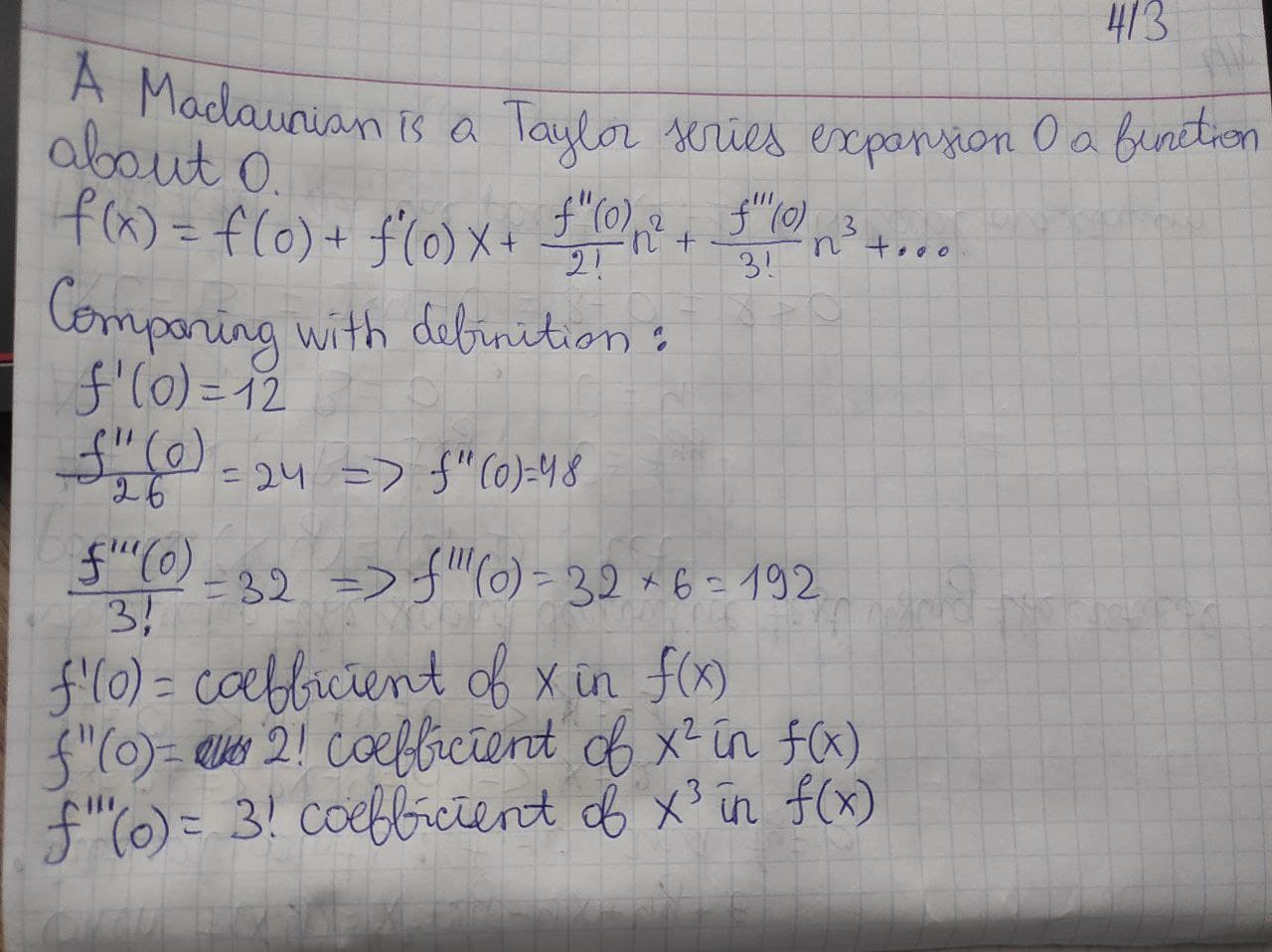# Let f be a function whose Maclaurin series expansion. f(x)=3+12x+24x^2+32x^3+... Explain how you can determine f'(0), f''(0), and f'''(0) simply by anUkusakazaL 2021-01-08 Answered
Let f be a function whose Maclaurin series expansion.
$f\left(x\right)=3+12x+24{x}^{2}+32{x}^{3}+\dots$
Explain how you can determine f'(0), f''(0), and f'''(0) simply by analyzing the oefficients of x, ${x}^{2}$, and ${x}^{3}$ in the given representation and without directly alculating f'(x), f''(x), and f'''(x) from the representation above.
You can still ask an expert for help

• Questions are typically answered in as fast as 30 minutes

Solve your problem for the price of one coffee

• Math expert for every subject
• Pay only if we can solve itNathanael Webber

A Macluarin series is a Taylor series expansion 0 a function about 0,
$f\left(x\right)=f\left(0\right)+{f}^{\prime }\left(0\right)x+\frac{f{}^{″}\left(0\right)}{2!}{n}^{2}+\frac{f{}^{‴}\left(0\right)}{3!}{n}^{3}+\dots$
Given that $f\left(x\right)=3+12x+24{x}^{2}+32{x}^{3}+..$.
Comparing with the above definition we get f'(0)12,
$\frac{f{}^{″}\left(0\right)}{26}=24⇒f{}^{″}\left(0\right)=48$
$\frac{f{}^{‴}\left(0\right)}{3!}=32⇒f{}^{‴}\left(0\right)=32\cdot 6=192$
f'(0)=coefficient of $x\in f\left(x\right)$
f''(0)=2! x coefficient of ${x}^{2}\in f\left(x\right)$
f'''(0)=3! x coefficient of ${x}^{3}\in f\left(x\right)$

###### Not exactly what you’re looking for?Jeffrey Jordon

Explanation is on photo: